Homework Help Question & Answers

# W The stream function « in a two-dimensional flow field is given as Q = 4x...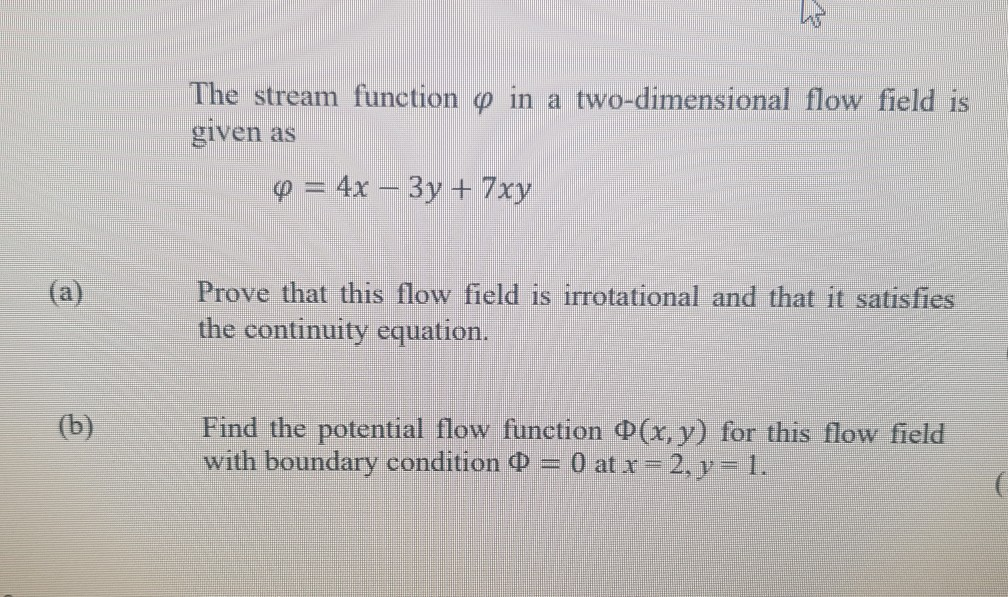W The stream function « in a two-dimensional flow field is given as Q = 4x – 3y + 7xy (a) Prove that this flow field is irrotational and that it satisfies the continuity equation. (b) Find the potential flow function 0(x, y) for this flow field with boundary condition Q = 0 at x = 2, y = 1.

#### Homework Answers

Answer #1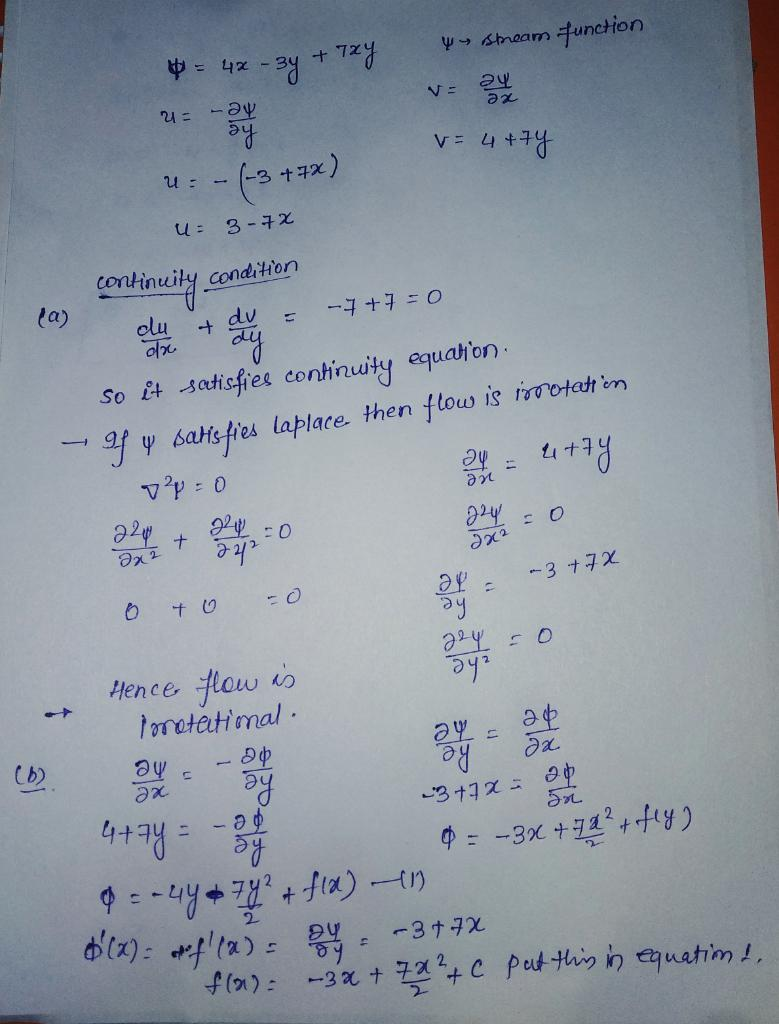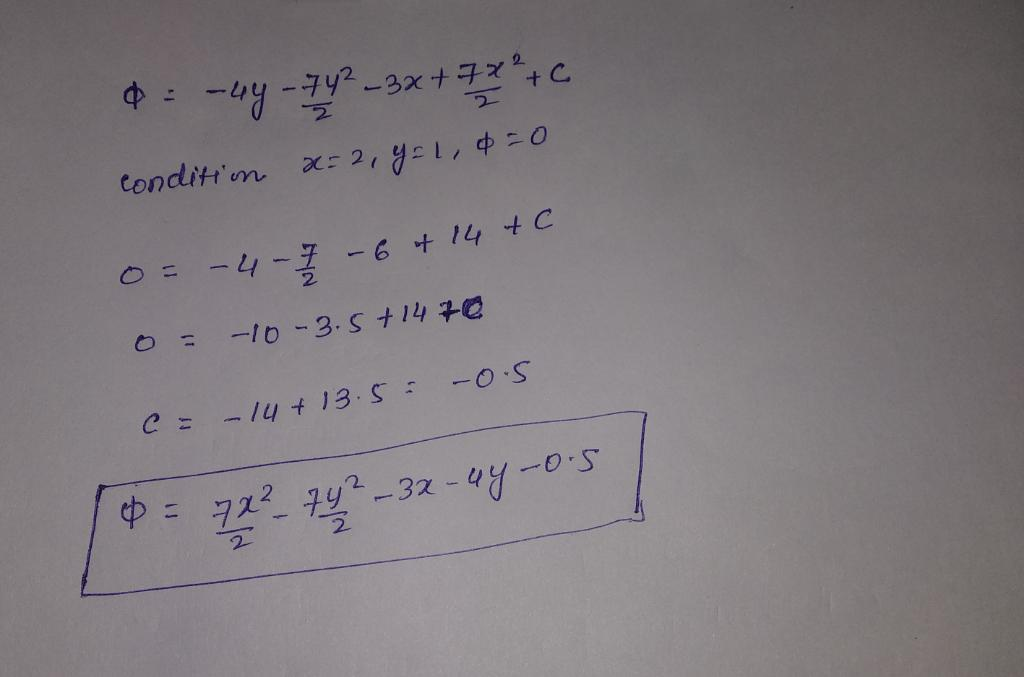Know the answer?
Your Answer:

#### Post as a guest

Your Name:

What's your source?

#### Earn Coin

Coins can be redeemed for fabulous gifts.

Not the answer you're looking for? Ask your own homework help question. Our experts will answer your question WITHIN MINUTES for Free.
Similar Homework Help Questions
• ### The stream function for an incompressible, two- dimensional flow field is v-ay-by where a and b...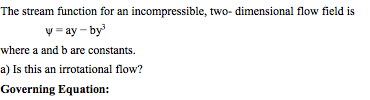The stream function for an incompressible, two- dimensional flow field is v-ay-by where a and b are constants. a) Is this an irrotational flow? Governing Equation:

• ### Q1. (a) The velocity components of a certain two-dimensional flow field are claimed to be given...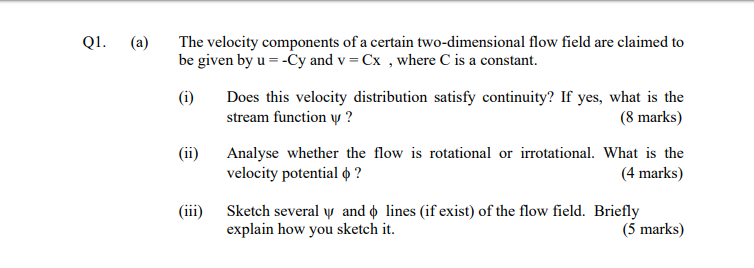Q1. (a) The velocity components of a certain two-dimensional flow field are claimed to be given by u = -Cy and v = Cx , where is a constant. (i) Does this velocity distribution satisfy continuity? If yes, what is the stream function y ? (8 marks) (ii) Analyse whether the flow is rotational or irrotational. What is the velocity potential o ? (4 marks) (iii) Sketch several y and lines (if exist) of the flow field. Briefly explain how...

• ### The stream function for a given two-dimensional flow field is w = 11x²y- (11/3)y3 Determine the...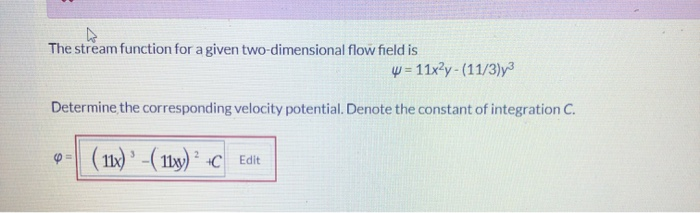The stream function for a given two-dimensional flow field is w = 11x²y- (11/3)y3 Determine the corresponding velocity potential. Denote the constant of integration C. 4- (11x) ' - ( 11x) +C Edie

• ### can you solve the last question (e) Q1. Consider a steady, two-dimensional, incompressible flow field has...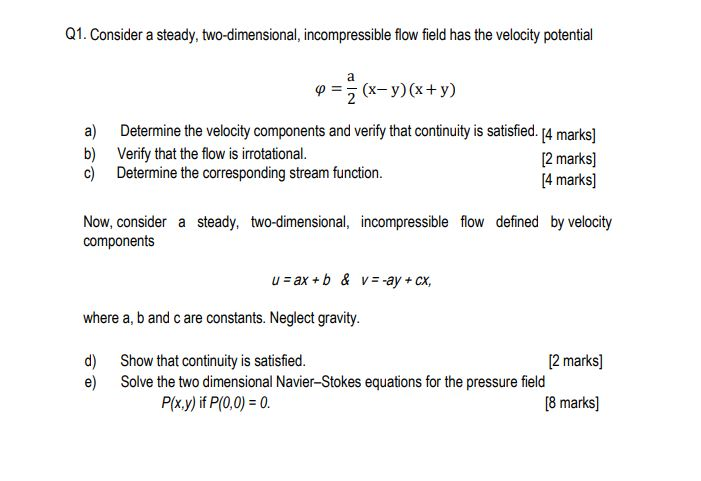can you solve the last question (e) Q1. Consider a steady, two-dimensional, incompressible flow field has the velocity potential a) b) c) 2 (x-7)(x+y) Determine the velocity components and verify that continuity is satisfied. [4 marks] Verify that the flow is irrotational. [2 marks] Determine the corresponding stream function. [4 marks) Now, consider a steady, two-dimensional, incompressible flow defined by velocity components u = ax + b&v=-ay + cx, where a, b and care constants. Neglect gravity. d) e) Show...

• ### The Velocity field of a flow is given by V=4xy^2i + 4x^2yj. a) calculate the stream...

The Velocity field of a flow is given by V=4xy^2i + 4x^2yj. a) calculate the stream function and the velocity potential b) find the equation for the stream line passing through point x=1, y=1. plot this function accurately c) find the equation for the equipotential line passing through point x=1, y=1. plot this function accurately on the same graph as part b.

• ### Incompressible fluid flow field 2. (a) An incompressible fluid flow field is given as Vx =...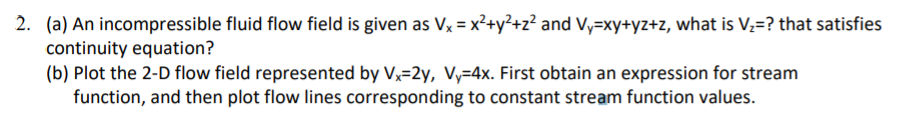Incompressible fluid flow field 2. (a) An incompressible fluid flow field is given as Vx = x2+y+z2 and Vy=xy+yz+z, what is V?=? that satisfies continuity equation? (b) Plot the 2-D flow field represented by Vx=2y, Vy=4x. First obtain an expression for stream function, and then plot flow lines corresponding to constant stream function values.

• ### Q1. (a) The velocity components of a certain two-dimensional flow field are claimed to be given...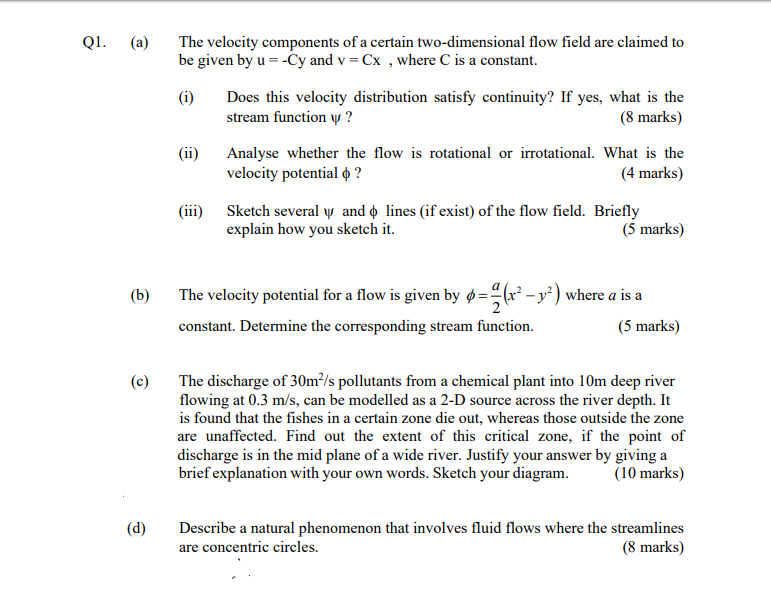Q1. (a) The velocity components of a certain two-dimensional flow field are claimed to be given by u =-Cy and v = Cx , where is a constant. (i) Does this velocity distribution satisfy continuity? If yes, what is the stream function y? (8 marks) (ii) Analyse whether the flow is rotational or irrotational. What is the velocity potential o ? (4 marks) (iii) Sketch several y and © lines (if exist) of the flow field. Briefly explain how you...

• ### In a steady-state two-dimensional heat flow problem, the temperature, u, at any point in the doma...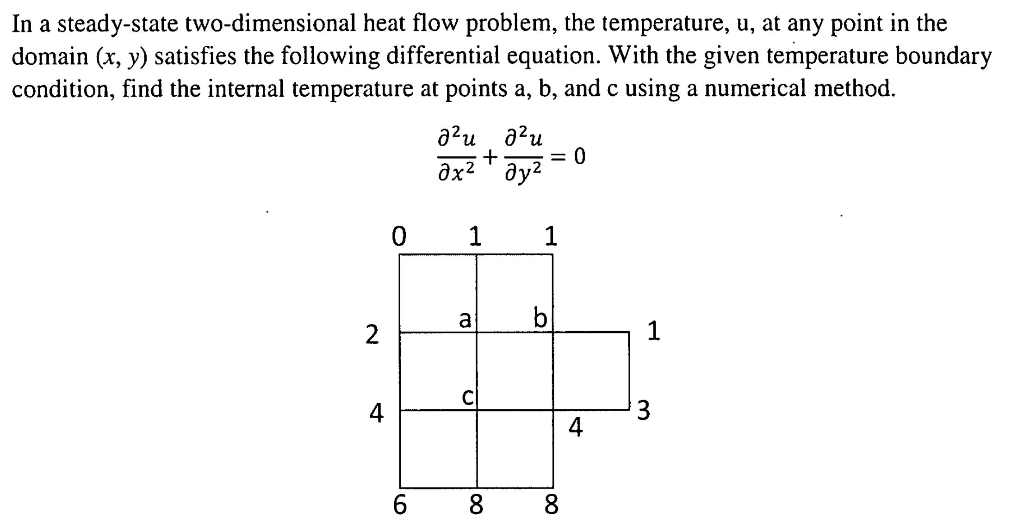In a steady-state two-dimensional heat flow problem, the temperature, u, at any point in the domain (x, y) satisfies the following differential equation. With the given temperature boundary condition, find the internal temperature at points a, b, and c using a numerical method. 0 4 4 In a steady-state two-dimensional heat flow problem, the temperature, u, at any point in the domain (x, y) satisfies the following differential equation. With the given temperature boundary condition, find the internal temperature at...

• ### The velocity in a certain two-dimensional flow field is given by the equation: ✓ = 2xti...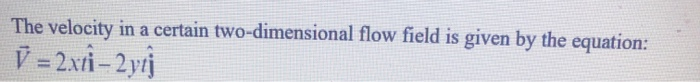The velocity in a certain two-dimensional flow field is given by the equation: ✓ = 2xti – 2 yı where the velocity is in ft/s when x, y, and t are in feet and seconds, respectively. (a) Is flow steady or unsteady (b) Determine the expression of acceleration (c) Check if the flow is compressible or incompressible (d) Check if the flow is rotational or irrotational (e) Sketch the streamlines of t= ls on a x-y plane W

• ### Question 1 [Total 20 marks] (a) [5 marks] In a steady-state two-dimensional heat flow problem, th...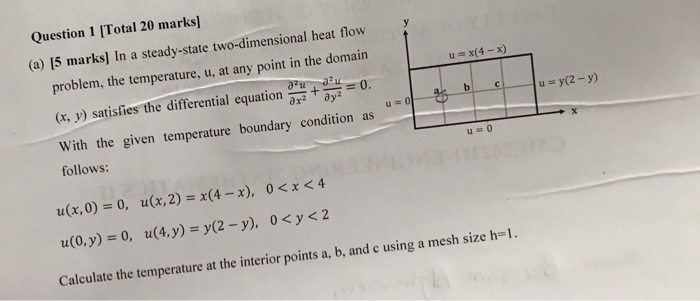Question 1 [Total 20 marks] (a) [5 marks] In a steady-state two-dimensional heat flow problem, the temperature, u, at any point in the domain (t, ) satisfies the differential equation u y(2-y) u= U0F With the given temperature boundary condition as follows: u(x, 0) = 0, u(x, 2) = x(4-x), 0 < x < 4 Calculate the temperature at the interior points a, b, and c using a mesh size h-1. Question 1 [Total 20 marks] (a) [5 marks] In...